# class mrpt::opengl::CPolyhedron

This class represents arbitrary polyhedra.

The class includes a set of static methods to create common polyhedrons. The class includes many methods to create standard polyhedra, not intended to be fast but to be simple. For example, the dodecahedron is not created efficiently: first, an icosahedron is created, and a duality operator is applied to it, which yields the dodecahedron. This way, code is much smaller, although much slower. This is not a big problem, since polyhedron creation does not usually take a significant amount of time (they are created once and rendered many times). Polyhedra information and models have been gotten from the Wikipedia, https://wikipedia.org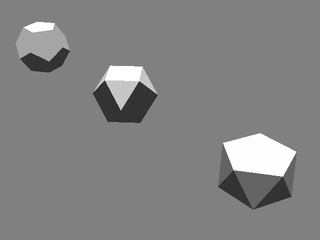opengl::COpenGLScene

#include <mrpt/opengl/CPolyhedron.h>

class CPolyhedron:
{
public:
// structs

struct TPolyhedronEdge;
struct TPolyhedronFace;

// construction

CPolyhedron();
CPolyhedron(const std::vector<mrpt::math::TPoint3D>& vertices, const std::vector<TPolyhedronFace>& faces, bool doCheck = true);
CPolyhedron(const std::vector<math::TPolygon3D>& polys);

CPolyhedron(
const std::vector<mrpt::math::TPoint3D>& vertices,
const std::vector<std::vector<uint32_t>>& faces
);

//
methods

virtual void render(const RenderContext& rc) const;
virtual void renderUpdateBuffers() const;
virtual void freeOpenGLResources();
virtual void onUpdateBuffers_Wireframe();
virtual void onUpdateBuffers_Triangles();
static CPolyhedron::Ptr CreateRhombicuboctahedron(double radius, bool type = true);
static CPolyhedron::Ptr CreateIcosidodecahedron(double radius, bool type = true);
static CPolyhedron::Ptr CreateCubicPrism(double x1, double x2, double y1, double y2, double z1, double z2);
static CPolyhedron::Ptr CreateCubicPrism(const mrpt::math::TPoint3D& p1, const mrpt::math::TPoint3D& p2);
static CPolyhedron::Ptr CreatePyramid(const std::vector<mrpt::math::TPoint2D>& baseVertices, double height);
static CPolyhedron::Ptr CreateDoublePyramid(const std::vector<mrpt::math::TPoint2D>& baseVertices, double height1, double height2);
static CPolyhedron::Ptr CreateTruncatedPyramid(const std::vector<mrpt::math::TPoint2D>& baseVertices, double height, double ratio);
static CPolyhedron::Ptr CreateFrustum(const std::vector<mrpt::math::TPoint2D>& baseVertices, double height, double ratio);
static CPolyhedron::Ptr CreateCustomPrism(const std::vector<mrpt::math::TPoint2D>& baseVertices, double height);

static CPolyhedron::Ptr CreateCustomAntiprism(
const std::vector<mrpt::math::TPoint2D>& bottomBase,
const std::vector<mrpt::math::TPoint2D>& topBase,
double height
);

static CPolyhedron::Ptr CreateParallelepiped(const mrpt::math::TPoint3D& base, const mrpt::math::TPoint3D& v1, const mrpt::math::TPoint3D& v2, const mrpt::math::TPoint3D& v3);

static CPolyhedron::Ptr CreateBifrustum(
const std::vector<mrpt::math::TPoint2D>& baseVertices,
double height1,
double ratio1,
double height2,
double ratio2
);

static CPolyhedron::Ptr CreateTrapezohedron(uint32_t numBaseEdges, double baseRadius, double basesDistance);
static CPolyhedron::Ptr CreateRegularAntiprism(uint32_t numBaseEdges, double baseRadius, double height);
static CPolyhedron::Ptr CreateRegularPrism(uint32_t numBaseEdges, double baseRadius, double height);
static CPolyhedron::Ptr CreateRegularPyramid(uint32_t numBaseEdges, double baseRadius, double height);

static CPolyhedron::Ptr CreateRegularDoublePyramid(
uint32_t numBaseEdges,
double height1,
double height2
);

static CPolyhedron::Ptr CreateArchimedeanRegularPrism(uint32_t numBaseEdges, double baseRadius);
static CPolyhedron::Ptr CreateArchimedeanRegularAntiprism(uint32_t numBaseEdges, double baseRadius);
static CPolyhedron::Ptr CreateRegularTruncatedPyramid(uint32_t numBaseEdges, double baseRadius, double height, double ratio);
static CPolyhedron::Ptr CreateRegularFrustum(uint32_t numBaseEdges, double baseRadius, double height, double ratio);

static CPolyhedron::Ptr CreateRegularBifrustum(
uint32_t numBaseEdges,
double height1,
double ratio1,
double height2,
double ratio2
);

static CPolyhedron::Ptr CreateCupola(uint32_t numBaseEdges, double edgeLength);
static CPolyhedron::Ptr CreateCatalanTrapezohedron(uint32_t numBaseEdges, double height);
static CPolyhedron::Ptr CreateCatalanDoublePyramid(uint32_t numBaseEdges, double height);

static CPolyhedron::Ptr CreateJohnsonSolidWithConstantBase(
uint32_t numBaseEdges,
const std::string& components,
size_t shifts = 0
);

CPolyhedron::Ptr getDual() const;
CPolyhedron::Ptr truncate(double factor) const;
CPolyhedron::Ptr cantellate(double factor) const;
CPolyhedron::Ptr augment(double height) const;
CPolyhedron::Ptr augment(double height, size_t numVertices) const;
CPolyhedron::Ptr augment(bool direction = false) const;
CPolyhedron::Ptr augment(size_t numVertices, bool direction = false) const;
CPolyhedron::Ptr rotate(double angle) const;
CPolyhedron::Ptr scale(double factor) const;
virtual mrpt::math::TBoundingBox getBoundingBox() const;
virtual bool traceRay(const mrpt::poses::CPose3D& o, double& dist) const;
void getVertices(std::vector<mrpt::math::TPoint3D>& vertices) const;
void getEdges(std::vector<TPolyhedronEdge>& edges) const;
void getFaces(std::vector<TPolyhedronFace>& faces) const;
uint32_t getNumberOfVertices() const;
uint32_t getNumberOfEdges() const;
uint32_t getNumberOfFaces() const;
void getEdgesLength(std::vector<double>& lengths) const;
void getFacesArea(std::vector<double>& areas) const;
double getVolume() const;
bool isWireframe() const;
void setWireframe(bool enabled = true);
void getSetOfPolygons(std::vector<math::TPolygon3D>& vec) const;
void getSetOfPolygonsAbsolute(std::vector<math::TPolygon3D>& vec) const;
bool isClosed() const;
void makeConvexPolygons();
void getCenter(mrpt::math::TPoint3D& center) const;
void updatePolygons() const;

void InitFromVertAndFaces(
const std::vector<mrpt::math::TPoint3D>& vertices,
const std::vector<TPolyhedronFace>& faces,
bool doCheck = true
);

template <class T>
static size_t getIntersection(const CPolyhedron::Ptr& p1, const CPolyhedron::Ptr& p2, T& container);

static CPolyhedron::Ptr CreateNoCheck(const std::vector<mrpt::math::TPoint3D>& vertices, const std::vector<TPolyhedronFace>& faces);
static CPolyhedron::Ptr CreateEmpty();
};

## Inherited Members

public:
// structs

struct RenderContext;

//
methods

virtual void render(const RenderContext& rc) const = 0;
virtual void renderUpdateBuffers() const = 0;
virtual auto getBoundingBox() const = 0;
virtual void freeOpenGLResources() = 0;
virtual void onUpdateBuffers_Wireframe() = 0;
virtual void onUpdateBuffers_Triangles() = 0;

## Construction

CPolyhedron()

Basic empty constructor.

CPolyhedron(
const std::vector<mrpt::math::TPoint3D>& vertices,
const std::vector<TPolyhedronFace>& faces,
bool doCheck = true
)

Basic constructor with a list of vertices and another of faces, checking for correctness.

## Methods

virtual void render(const RenderContext& rc) const

Implements the rendering of 3D objects in each class derived from CRenderizable.

This can be called more than once (one per required shader program) if the object registered several shaders.

renderUpdateBuffers

virtual void renderUpdateBuffers() const

Called whenever m_outdatedBuffers is true: used to re-generate OpenGL vertex buffers, etc.

before they are sent for rendering in render()

virtual void freeOpenGLResources()

Free opengl buffers.

virtual shader_list_t requiredShaders() const

Returns the ID of the OpenGL shader program required to render this class.

virtual void onUpdateBuffers_Wireframe()

Must be implemented in derived classes to update the geometric entities to be drawn in “m_*_buffer” fields.

virtual void onUpdateBuffers_Triangles()

Must be implemented in derived classes to update the geometric entities to be drawn in “m_*_buffer” fields.

static CPolyhedron::Ptr CreateTetrahedron(double radius)

Creates a regular tetrahedron (see http://en.wikipedia.org/wiki/Tetrahedron).

The tetrahedron is created as a triangular pyramid whose edges and vertices are transitive. The tetrahedron is the dual to itself.static CPolyhedron::Ptr CreateHexahedron(double radius)

Creates a regular cube, also called hexahedron (see http://en.wikipedia.org/wiki/Hexahedron).

The hexahedron is created as a cubic prism which transitive edges. Another ways to create it include:

• Dual to an octahedron.

• Parallelepiped with three orthogonal, equally-lengthed vectors.

• Triangular trapezohedron with proper height.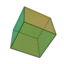static CPolyhedron::Ptr CreateOctahedron(double radius)

Creates a regular octahedron (see http://en.wikipedia.org/wiki/Octahedron).

The octahedron is created as a square bipyramid whit transitive edges and vertices. Another ways to create an octahedron are:

• Dual to an hexahedron

• Triangular antiprism with transitive vertices.

• Conveniently truncated tetrahedron.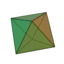static CPolyhedron::Ptr CreateDodecahedron(double radius)

Creates a regular dodecahedron (see http://en.wikipedia.org/wiki/Dodecahedron).

The dodecahedron is created as the dual to an icosahedron.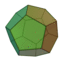static CPolyhedron::Ptr CreateIcosahedron(double radius)

Creates a regular icosahedron (see http://en.wikipedia.org/wiki/Icosahedron).

The icosahedron is created as a gyroelongated pentagonal bipyramid with transitive edges, and it’s the dual to a dodecahedron.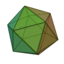static CPolyhedron::Ptr CreateTruncatedTetrahedron(double radius)

Creates a truncated tetrahedron, consisting of four triangular faces and for hexagonal ones (see http://en.wikipedia.org/wiki/Truncated_tetrahedron).

Its dual is the triakis tetrahedron.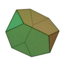static CPolyhedron::Ptr CreateCuboctahedron(double radius)

Creates a cuboctahedron, consisting of six square faces and eight triangular ones (see http://en.wikipedia.org/wiki/Cuboctahedron).

There are several ways to create a cuboctahedron:

• Hexahedron truncated to a certain extent.

• Octahedron truncated to a certain extent.

• Cantellated tetrahedron

• Dual to a rhombic dodecahedron.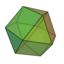static CPolyhedron::Ptr CreateTruncatedHexahedron(double radius)

Creates a truncated hexahedron, with six octogonal faces and eight triangular ones (see http://en.wikipedia.org/wiki/Truncated_hexahedron).

The truncated octahedron is dual to the triakis octahedron.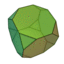static CPolyhedron::Ptr CreateTruncatedOctahedron(double radius)

Creates a truncated octahedron, with eight hexagons and eight squares (see http://en.wikipedia.org/wiki/Truncated_octahedron).

It’s the dual to the tetrakis hexahedron.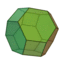static CPolyhedron::Ptr CreateRhombicuboctahedron(double radius, bool type = true)

Creates a rhombicuboctahedron, with 18 squares and 8 triangles (see http://en.wikipedia.org/wiki/Rhombicuboctahedron), calculated as an elongated square bicupola.

It can also be calculated as a cantellated hexahedron or octahedron, and its dual is the deltoidal icositetrahedron. If the second argument is set to false, the lower cupola is rotated, so that the objet created is an elongated square gyrobicupola (see http://en.wikipedia.org/wiki/Elongated_square_gyrobicupola). This is not an archimedean solid, but a Johnson one, since it hasn’t got vertex transitivity.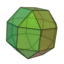static CPolyhedron::Ptr CreateIcosidodecahedron(double radius, bool type = true)

Creates an icosidodecahedron, with 12 pentagons and 20 triangles (see http://en.wikipedia.org/wiki/Icosidodecahedron).

Certain truncations of either a dodecahedron or an icosahedron yield an icosidodecahedron. The dual of the icosidodecahedron is the rhombic triacontahedron. If the second argument is set to false, the lower rotunda is rotated. In this case, the object created is a pentagonal orthobirotunda (see http://en.wikipedia.org/wiki/Pentagonal_orthobirotunda). This object presents symmetry against the XY plane and is not vertex transitive, so it’s a Johnson’s solid.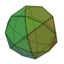static CPolyhedron::Ptr CreateTruncatedDodecahedron(double radius)

Creates a truncated dodecahedron, consisting of 12 dodecagons and 20 triangles (see http://en.wikipedia.org/wiki/Truncated_dodecahedron).

The truncated dodecahedron is the dual to the triakis icosahedron.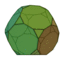static CPolyhedron::Ptr CreateTruncatedIcosahedron(double radius)

Creates a truncated icosahedron, consisting of 20 hexagons and 12 pentagons.

This object resembles a typical soccer ball (see http://en.wikipedia.org/wiki/Truncated_icosahedron). The pentakis dodecahedron is the dual to the truncated icosahedron.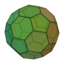static CPolyhedron::Ptr CreateRhombicosidodecahedron(double radius)

Creates a rhombicosidodecahedron, consisting of 30 squares, 12 pentagons and 20 triangles (see http://en.wikipedia.org/wiki/Rhombicosidodecahedron).

This object can be obtained as the cantellation of either a dodecahedron or an icosahedron. The dual of the rhombicosidodecahedron is the deltoidal hexecontahedron.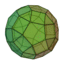static CPolyhedron::Ptr CreatePentagonalRotunda(double radius)

Creates a pentagonal rotunda (half an icosidodecahedron), consisting of six pentagons, ten triangles and a decagon (see http://en.wikipedia.org/wiki/Pentagonal_rotunda).

static CPolyhedron::Ptr CreateTriakisTetrahedron(double radius)

Creates a triakis tetrahedron, dual to the truncated tetrahedron.

This body consists of 12 isosceles triangles (see http://en.wikipedia.org/wiki/Triakis_tetrahedron).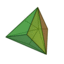CreateTruncatedTetrahedron

static CPolyhedron::Ptr CreateRhombicDodecahedron(double radius)

Creates a rhombic dodecahedron, dual to the cuboctahedron.

This body consists of 12 rhombi (see http://en.wikipedia.org/wiki/Rhombic_dodecahedron).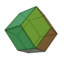CreateCuboctahedron

static CPolyhedron::Ptr CreateTriakisOctahedron(double radius)

Creates a triakis octahedron, dual to the truncated hexahedron.

This body consists of 24 isosceles triangles (see http://en.wikipedia.org/wiki/Triakis_octahedron).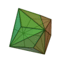CreateTruncatedHexahedron

static CPolyhedron::Ptr CreateTetrakisHexahedron(double radius)

Creates a tetrakis hexahedron, dual to the truncated octahedron.

This body consists of 24 isosceles triangles (see http://en.wikipedia.org/wiki/Tetrakis_hexahedron).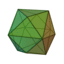CreateTruncatedOctahedron

static CPolyhedron::Ptr CreateDeltoidalIcositetrahedron(double radius)

Creates a deltoidal icositetrahedron, dual to the rhombicuboctahedron.

This body consists of 24 kites (see http://en.wikipedia.org/wiki/Deltoidal_icositetrahedron).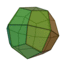CreateRhombicuboctahedron

static CPolyhedron::Ptr CreateRhombicTriacontahedron(double radius)

Creates a rhombic triacontahedron, dual to the icosidodecahedron.

This body consists of 30 rhombi (see http://en.wikipedia.org/wiki/Rhombic_triacontahedron).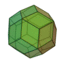CreateIcosidodecahedron

static CPolyhedron::Ptr CreateTriakisIcosahedron(double radius)

Creates a triakis icosahedron, dual to the truncated dodecahedron.

This body consists of 60 isosceles triangles http://en.wikipedia.org/wiki/Triakis_icosahedron).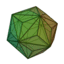CreateTruncatedDodecahedron

static CPolyhedron::Ptr CreatePentakisDodecahedron(double radius)

Creates a pentakis dodecahedron, dual to the truncated icosahedron.

This body consists of 60 isosceles triangles (see http://en.wikipedia.org/wiki/Pentakis_dodecahedron).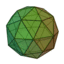CreateTruncatedIcosahedron

static CPolyhedron::Ptr CreateDeltoidalHexecontahedron(double radius)

Creates a deltoidal hexecontahedron, dual to the rhombicosidodecahedron.

This body consists of 60 kites (see http://en.wikipedia.org/wiki/Deltoidal_hexecontahedron).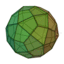CreateRhombicosidodecahedron

static CPolyhedron::Ptr CreateCubicPrism(
double x1,
double x2,
double y1,
double y2,
double z1,
double z2
)

Creates a cubic prism, given the coordinates of two opposite vertices.

Each edge will be parallel to one of the coordinate axes, although the orientation may change by assigning a pose to the object.

static CPolyhedron::Ptr CreateCubicPrism(const mrpt::math::TPoint3D& p1, const mrpt::math::TPoint3D& p2)

Creates a cubic prism, given two opposite vertices.

static CPolyhedron::Ptr CreatePyramid(const std::vector<mrpt::math::TPoint2D>& baseVertices, double height)

Creates a custom pyramid, using a set of 2D vertices which will lie on the XY plane.

static CPolyhedron::Ptr CreateDoublePyramid(
const std::vector<mrpt::math::TPoint2D>& baseVertices,
double height1,
double height2
)

Creates a double pyramid, using a set of 2D vertices which will lie on the XY plane.

The second height is used with the downwards pointing pyramid, so that it will effectively point downwards if it’s positive.

static CPolyhedron::Ptr CreateTruncatedPyramid(
const std::vector<mrpt::math::TPoint2D>& baseVertices,
double height,
double ratio
)

Creates a truncated pyramid, using a set of vertices which will lie on the XY plane.

Do not try to use with a ratio equal to zero; use CreatePyramid instead. When using a ratio of 1, it will create a Prism.

static CPolyhedron::Ptr CreateFrustum(
const std::vector<mrpt::math::TPoint2D>& baseVertices,
double height,
double ratio
)

This is a synonym for CreateTruncatedPyramid.

CreateTruncatedPyramid

static CPolyhedron::Ptr CreateCustomPrism(const std::vector<mrpt::math::TPoint2D>& baseVertices, double height)

Creates a custom prism with vertical edges, given any base which will lie on the XY plane.

static CPolyhedron::Ptr CreateCustomAntiprism(
const std::vector<mrpt::math::TPoint2D>& bottomBase,
const std::vector<mrpt::math::TPoint2D>& topBase,
double height
)

Creates a custom antiprism, using two custom bases.

For better results, the top base should be slightly rotated with respect to the bottom one.

static CPolyhedron::Ptr CreateParallelepiped(const mrpt::math::TPoint3D& base, const mrpt::math::TPoint3D& v1, const mrpt::math::TPoint3D& v2, const mrpt::math::TPoint3D& v3)

Creates a parallelepiped, given a base point and three vectors represented as points.

CreateCubicPrism

static CPolyhedron::Ptr CreateBifrustum(
const std::vector<mrpt::math::TPoint2D>& baseVertices,
double height1,
double ratio1,
double height2,
double ratio2
)

Creates a bifrustum, or double truncated pyramid, given a base which will lie on the XY plane.

static CPolyhedron::Ptr CreateTrapezohedron(
uint32_t numBaseEdges,
double basesDistance
)

Creates a trapezohedron, consisting of 2*N kites, where N is the number of edges in the base.

The base radius controls the polyhedron height, whilst the distance between bases affects the height. When the number of edges equals 3, the polyhedron is actually a parallelepiped, and it can even be a cube.

static CPolyhedron::Ptr CreateRegularAntiprism(
uint32_t numBaseEdges,
double height
)

Creates an antiprism whose base is a regular polygon.

The upper base is rotated $$\frac\pi N$$ with respect to the lower one, where N is the number of vertices in the base, and thus the lateral triangles are isosceles.

static CPolyhedron::Ptr CreateRegularPrism(
uint32_t numBaseEdges,
double height
)

Creates a regular prism whose base is a regular polygon and whose edges are either parallel or perpendicular to the XY plane.

static CPolyhedron::Ptr CreateRegularPyramid(
uint32_t numBaseEdges,
double height
)

Creates a regular pyramid whose base is a regular polygon.

CreatePyramid

static CPolyhedron::Ptr CreateRegularDoublePyramid(
uint32_t numBaseEdges,
double height1,
double height2
)

Creates a regular double pyramid whose base is a regular polygon.

CreateDoublePyramid

static CPolyhedron::Ptr CreateArchimedeanRegularPrism(uint32_t numBaseEdges, double baseRadius)

Creates a regular prism whose lateral area is comprised of squares, and so each face of its is a regular polygon.

Due to vertex transitivity, the resulting object is always archimedean.

static CPolyhedron::Ptr CreateArchimedeanRegularAntiprism(
uint32_t numBaseEdges,
)

Creates a regular antiprism whose lateral polygons are equilateral triangles, and so each face of its is a regular polygon.

Due to vertex transitivity, the resulting object is always archimedean.

static CPolyhedron::Ptr CreateRegularTruncatedPyramid(
uint32_t numBaseEdges,
double height,
double ratio
)

Creates a regular truncated pyramid whose base is a regular polygon.

CreateTruncatedPyramid

static CPolyhedron::Ptr CreateRegularFrustum(
uint32_t numBaseEdges,
double height,
double ratio
)

This is a synonym for CreateRegularTruncatedPyramid.

CreateRegularTruncatedPyramid

static CPolyhedron::Ptr CreateRegularBifrustum(
uint32_t numBaseEdges,
double height1,
double ratio1,
double height2,
double ratio2
)

Creates a bifrustum (double truncated pyramid) whose base is a regular polygon lying in the XY plane.

CreateBifrustum

static CPolyhedron::Ptr CreateCupola(uint32_t numBaseEdges, double edgeLength)

Creates a cupola.

Parameters:

 std::logic_error if the number of edges is odd or less than four.
static CPolyhedron::Ptr CreateCatalanTrapezohedron(uint32_t numBaseEdges, double height)

Creates a trapezohedron whose dual is exactly an archimedean antiprism.

Creates a cube if numBaseEdges is equal to 3. Todo Actually resulting height is significantly higher than that passed to the algorithm.

static CPolyhedron::Ptr CreateCatalanDoublePyramid(uint32_t numBaseEdges, double height)

Creates a double pyramid whose dual is exactly an archimedean prism.

Creates an octahedron if numBaseEdges is equal to 4. Todo Actually resulting height is significantly higher than that passed to the algorithm.

static CPolyhedron::Ptr CreateJohnsonSolidWithConstantBase(
uint32_t numBaseEdges,
const std::string& components,
size_t shifts = 0
)

Creates a series of concatenated solids (most of which are prismatoids) whose base is a regular polygon with a given number of edges.

Every face of the resulting body will be a regular polygon, so it is a Johnson solid; in special cases, it may be archimedean or even platonic. The shape of the body is defined by the string argument, which can include one or more of the following: === ================================= =================================================================================

 P+ Upward pointing pyramid Must be the last object, vertex number cannot surpass 5 P- Downward pointing pyramid Must be the first object, vertex number cannot surpass 5 C+ Upward pointing cupola Must be the last object, vertex number must be an even number in the range 4-10. C- Downward pointing cupola Must be the first object, vertex number must be an even number in the range 4-10. GC+ Upward pointing cupola, rotated Must be the last object, vertex number must be an even number in the range 4-10. GC- Downward pointing cupola, rotated Must be the first object, vertex number must be an even number in the range 4-10. PR Archimedean prism Cannot abut other prism A Archimedean antiprism None R+ Upward pointing rotunda Must be the last object, vertex number must be exactly 10 R- Downward pointing rotunda Must be the first object, vertex number must be exactly 10 GR+ Upward pointing rotunda, rotated Must be the last object, vertex number must be exactly 10 GR- Downward pointing rotunda Must be the first object, vertex number must be exactly 10

Some examples of bodies are: ====== == ===================

 P+ 3 Tetrahedron PR 4 Hexahedron P-P+ 4 Octahedron A 3 Octahedron C+PRC- 8 Rhombicuboctahedron P-AP+ 5 Icosahedron R-R+ 10 Icosidodecahedron
CPolyhedron::Ptr getDual() const

Given a polyhedron, creates its dual.

Parameters:

 std::logic_error Can’t get the dual to this polyhedron.

CPolyhedron::Ptr truncate(double factor) const

Truncates a polyhedron to a given factor.

Parameters:

 std::logic_error Polyhedron truncation results in skew polygons and thus it’s impossible to perform.

CPolyhedron::Ptr cantellate(double factor) const

Cantellates a polyhedron to a given factor.

CPolyhedron::Ptr augment(double height) const

Augments a polyhedron to a given height.

This operation is roughly dual to the truncation: given a body P, the operation dtdP and aP yield resembling results.

CPolyhedron::Ptr augment(double height, size_t numVertices) const

Augments a polyhedron to a given height.

This method only affects to faces with certain number of vertices.

augment(double) const

CPolyhedron::Ptr augment(bool direction = false) const

Augments a polyhedron, so that the resulting triangles are equilateral.

If the argument is true, triangles are “cut” from the polyhedron, instead of being added.

Parameters:

 std::logic_error a non-regular face has been found.

augment(double) const

CPolyhedron::Ptr augment(size_t numVertices, bool direction = false) const

Augments a polyhedron, so that the resulting triangles are equilateral; affects only faces with certain number of faces.

If the second argument is true, triangles are “cut” from the polyhedron.

Parameters:

 std::logic_error a non-regular face has been found.

augment(double) const

CPolyhedron::Ptr rotate(double angle) const

Rotates a polyhedron around the Z axis a given amount of radians.

In some cases, this operation may be necessary to view the symmetry between related objects.

scale

CPolyhedron::Ptr scale(double factor) const

Scales a polyhedron to a given factor.

Parameters:

 std::logic_error factor is not a strictly positive number.

rotate

virtual mrpt::math::TBoundingBox getBoundingBox() const

Evaluates the bounding box of this object (including possible children) in the coordinate frame of the object parent.

virtual bool traceRay(const mrpt::poses::CPose3D& o, double& dist) const

Simulation of ray-trace, given a pose.

Returns true if the ray effectively collisions with the object (returning the distance to the origin of the ray in “dist”), or false in other case. “dist” variable yields undefined behaviour when false is returned

void getVertices(std::vector<mrpt::math::TPoint3D>& vertices) const

Gets a list with the polyhedron’s vertices.

void getEdges(std::vector<TPolyhedronEdge>& edges) const

Gets a list with the polyhedron’s edges.

void getFaces(std::vector<TPolyhedronFace>& faces) const

Gets a list with the polyhedron’s faces.

uint32_t getNumberOfVertices() const

Gets the amount of vertices.

uint32_t getNumberOfEdges() const

Gets the amount of edges.

uint32_t getNumberOfFaces() const

Gets the amount of faces.

void getEdgesLength(std::vector<double>& lengths) const

Gets a vector with each edge’s length.

void getFacesArea(std::vector<double>& areas) const

Gets a vector with each face’s area.

Won’t work properly if the polygons are not convex.

double getVolume() const

Gets the polyhedron volume.

Won’t work properly if the polyhedron is not convex.

bool isWireframe() const

Returns whether the polyhedron will be rendered as a wireframe object.

void setWireframe(bool enabled = true)

Sets whether the polyhedron will be rendered as a wireframe object.

void getSetOfPolygons(std::vector<math::TPolygon3D>& vec) const

Gets the polyhedron as a set of polygons.

mrpt::math::TPolygon3D

void getSetOfPolygonsAbsolute(std::vector<math::TPolygon3D>& vec) const

Gets the polyhedron as a set of polygons, with the pose transformation already applied.

bool isClosed() const

Returns true if the polygon is a completely closed object.

void makeConvexPolygons()

Recomputes polygons, if necessary, so that each one is convex.

void getCenter(mrpt::math::TPoint3D& center) const

Gets the center of the polyhedron.

void updatePolygons() const

Updates the mutable list of polygons used in rendering and ray tracing.

template <class T>
static size_t getIntersection(
const CPolyhedron::Ptr& p1,
const CPolyhedron::Ptr& p2,
T& container
)

Gets the intersection of two polyhedra, either as a set or as a matrix of intersections.

Each intersection is represented by a TObject3D.

mrpt::math::TObject3D

static CPolyhedron::Ptr CreateRandomPolyhedron(double radius)

Creates a random polyhedron from the static methods.

static CPolyhedron::Ptr CreateNoCheck(const std::vector<mrpt::math::TPoint3D>& vertices, const std::vector<TPolyhedronFace>& faces)

Creates a polyhedron without checking its correctness.

static CPolyhedron::Ptr CreateEmpty()

Creates an empty Polyhedron.